SSC BOARD PAPERS IMPORTANT TOPICS COVERED FOR BOARD EXAM 2024

### Balbharati solutions for Chemistry 12th Standard HSC for Maharashtra State Board chapter 3 - Ionic Equilibria

Exercises [Pages 61 - 62]

### Balbharati solutions for Chemistry 12th Standard HSC for Maharashtra State Board Chapter 3 Ionic Equilibria Exercises [Pages 61 - 62]

Exercises | Q 1.1 | Page 61

The pH of 10-8 M of HCl is ____________

• 8

• 7

• less than 7

• greater than 7

SOLUTION

The pH of 10-8 M of HCl is less than 7.

Concept: The pH Scale

Exercises | Q 1.2 | Page 61

Which of the following solution will have a pH value equal to 1.0?

• 50 mL of 0.1M HCl + 50mL of 0.1M NaOH

• 60 mL of 0.1M HCl + 40mL of 0.1M NaOH

• 20 mL of 0.1M HCl + 80mL of 0.1M NaOH

• 75 mL of 0.2M HCl + 25mL of 0.2M NaOH

SOLUTION

75 mL of 0.2M HCl + 25mL of 0.2M NaOH

Concept: The pH Scale

Exercises | Q 1.3 | Page 61

Which of the following is a buffer solution?

• CH3COONa + NaCl in water

• CH3COOH + HCl in water

• CH3COOH + CH3COONa in water

• HCl + NH4Cl in water

SOLUTION

CH3COOH + CH3COONa in water

Concept: Buffer Solutions

Exercises | Q 1.4 | Page 61

Choose the most correct answer :

The solubility product of a sparingly soluble salt AX is 5.2 × 10-13. Its solubility in mol dm-3 is ______.

• 7.2 × 10-7

• 1.35 × 10-4

• 7.2 × 10-8

• 13.5 × 10-8

SOLUTION

The solubility product of a sparingly soluble salt AX is 5.2 × 10-13. Its solubility in mol dm-3 is 7.2 × 10-7.

Concept: Solubility Product - Solubility product

Exercises | Q 1.5 | Page 61

Choose the most correct answer :

Blood in the human body is highly buffered at a pH of ________.

• 7.4

• 7.0

• 6.9

• 8.1

SOLUTION

Blood in the human body is highly buffered at a pH of 7.4.

Concept: The pH Scale

Exercises | Q 1.6 | Page 61

Choose the most correct answer :

The conjugate base of [Zn(H2O)4]2⊕ is __________.

• [Zn(H2O)4]2-NH3

• [Zn(H2O)3]2-

• [Zn(H2O)3OH]

• [Zn(H2O)H]3⊕

SOLUTION

The conjugate base of [Zn(H2O)4]2⊕ is [Zn(H2O)3OH].

Concept: Acids and Bases

Exercises | Q 1.7 | Page 61

Choose the most correct answer :

For pH > 7 the hydronium ion concentration would be _________.

• 10-7M

• < 10-7M

• > 10-7M

• ≥ 10-7M

SOLUTION

For pH > 7 the hydronium ion concentration would be < 10-7M .

Concept: The pH Scale

Exercises | Q 2.01 | Page 61

Answer the following in one sentence :

Why cations are Lewis acids?

SOLUTION

Cations are electron-deficient species and can accept an electron pair. Hence, cations are Lewis acids.

Exercises | Q 2.02 | Page 61

Answer the following in one sentence :

Why is KCl solution neutral to litmus?

SOLUTION

KCl, being salt of a strong acid (HCl) and a strong base (KOH), does not undergo hydrolysis. Hence, the KCl solution is neutral to litmus.

Exercises | Q 2.03 | Page 61

Answer the following in one sentence :

How are basic buffer solutions prepared?

SOLUTION

Basic buffer solutions are prepared by mixing aqueous solutions of a weak base and its salt with strong acid.

Exercises | Q 2.04 | Page 61

Answer the following in one sentence :

The dissociation constant of acetic acid is 1.8 × 10-5. Calculate percent dissociation of acetic acid in 0.01 M solution.

SOLUTION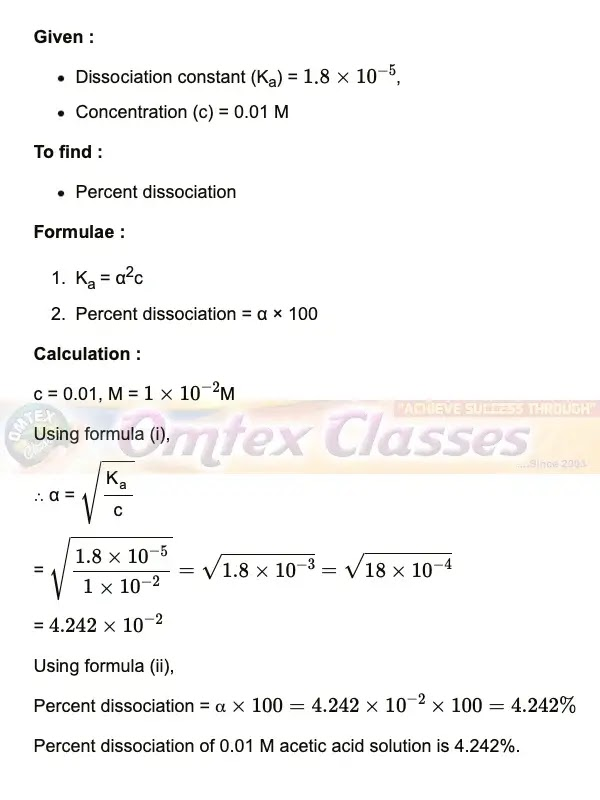Exercises | Q 2.05 | Page 61

Answer the following in one sentence :

Write one property of a buffer solution.

SOLUTION

Properties of buffer solution:

i. When a small amount of strong acid (or strong base) is added to a buffer solution, there is no significant change in the value of pH.

ii. The pH of a buffer solution is independent of the volume of the solution. Hence, the dilution of a buffer solution will not change its pH.

iii. The pH of a buffer solution does not change even if it is kept for a long time.

Exercises | Q 2.06 | Page 61

Answer the following in one sentence :

The pH of a solution is 6.06. Calculate its H ion concentration.

SOLUTION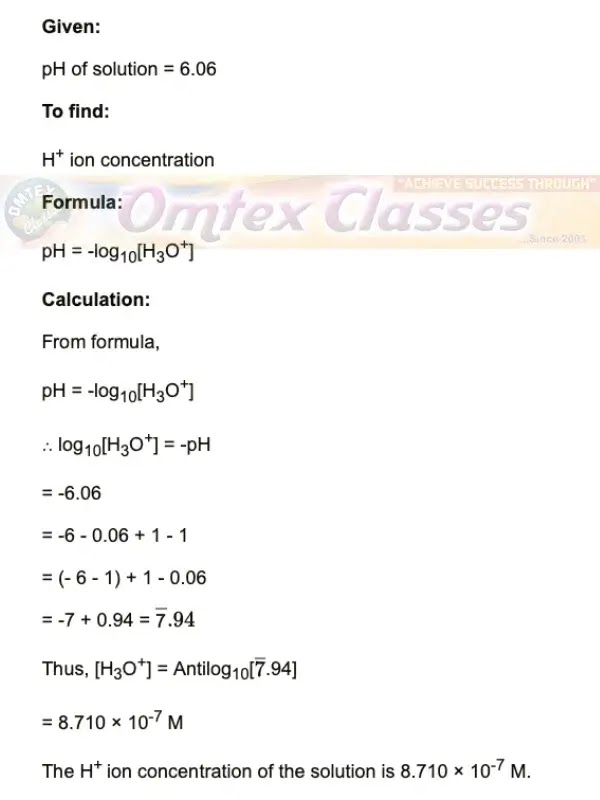Exercises | Q 2.07 | Page 61

Answer the following in one sentence :

Calculate the pH of 0.01 M sulphuric acid.

SOLUTION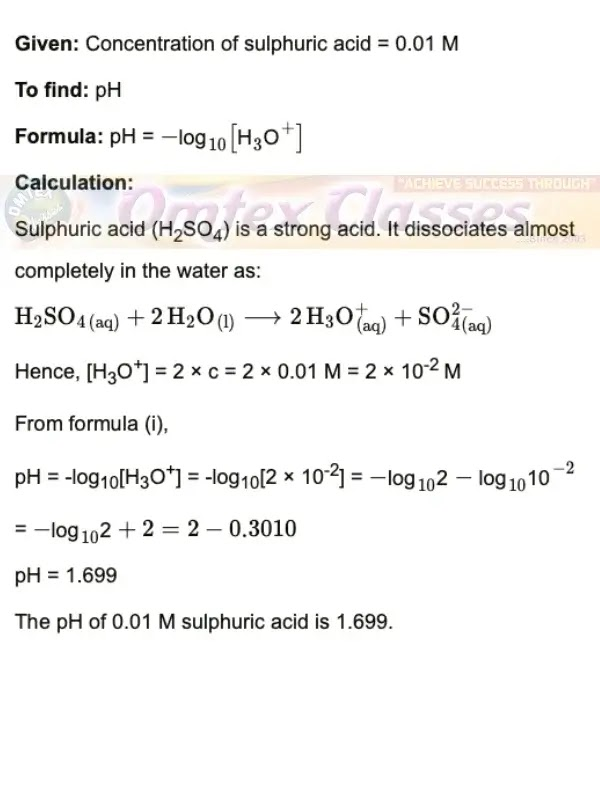Exercises | Q 2.08 | Page 61

Answer the following in one sentence :

The dissociation of H2S is suppressed in the presence of HCl. Name the phenomenon.

SOLUTION

The phenomenon due to which dissociation of H2S is suppressed in the presence of HCl is known as the common ion effect.

Exercises | Q 2.09 | Page 61

Answer the following in one sentence :

Why is it necessary to add H2SO4 while preparing the solution of CuSO4?

SOLUTION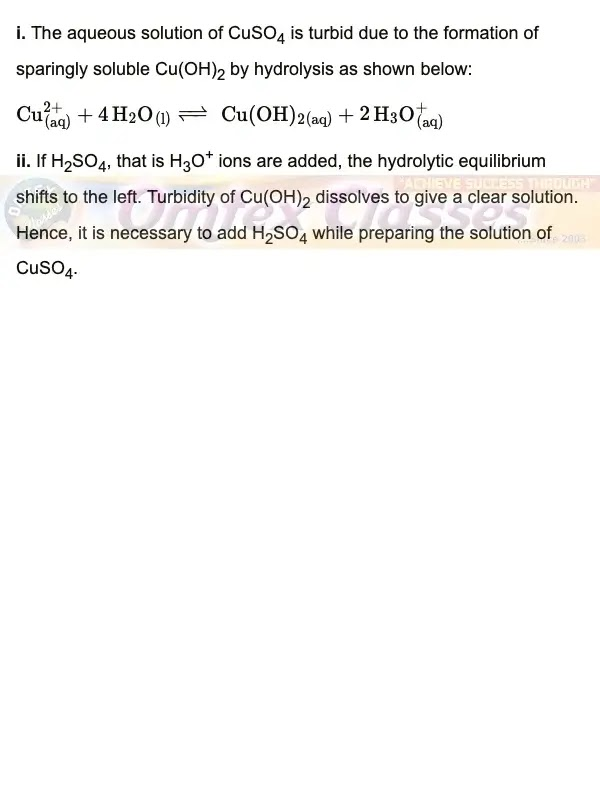Exercises | Q 2.1 | Page 61

Answer the following in one sentence :

Classify the following buffers into different types :

CH3COOH + CH3COONa

• Acidic buffer

• Basic buffer

SOLUTION

CH3COOH + CH3COONa - Acidic buffer

Exercises | Q 2.1 | Page 61

Answer the following in one sentence :

Classify the following buffers into different types :

NH4OH + NH4Cl

• Acidic buffer

• Basic buffer

SOLUTION

NH4OH + NH4Cl - Basic buffer

Exercises | Q 2.1 | Page 61

Answer the following in one sentence :

Classify the following buffers into different types :

Sodium benzoate + benzoic acid

• Acidic buffer

• Basic buffer

SOLUTION

Sodium benzoate + benzoic acid - Acidic buffer

Exercises | Q 2.1 | Page 61

Answer the following in one sentence :

Classify the following buffers into different types :

Cu(OH)2 + CuCl2

• Acidic buffer

• Basic buffer

SOLUTION

Cu(OH)2 + CuCl2 - Basic buffer

Exercises | Q 3.01 | Page 62

Answer the following in brief :

What are acids and bases according to Arrhenius theory?

SOLUTION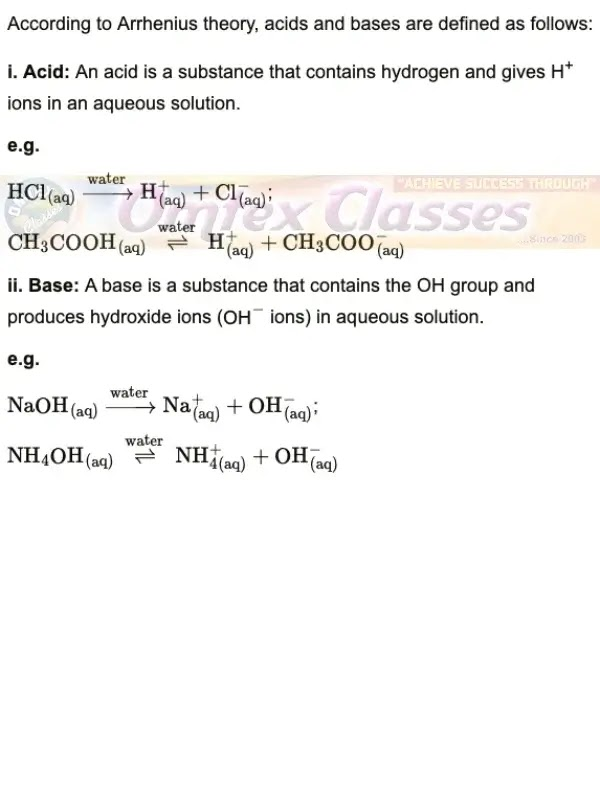Exercises | Q 3.02 | Page 62

Answer the following in brief :

What is meant by conjugate acid-base pair?

SOLUTION

i. The base produced by accepting the proton from acid is the conjugate base of that acid.

ii. Similarly, the acid produced when a base accepts a proton is called the conjugate acid of that base.

iii. A pair of an acid and a base differing by a proton is said to be a conjugate acid-base pair.

Exercises | Q 3.03 | Page 62

Answer the following in brief :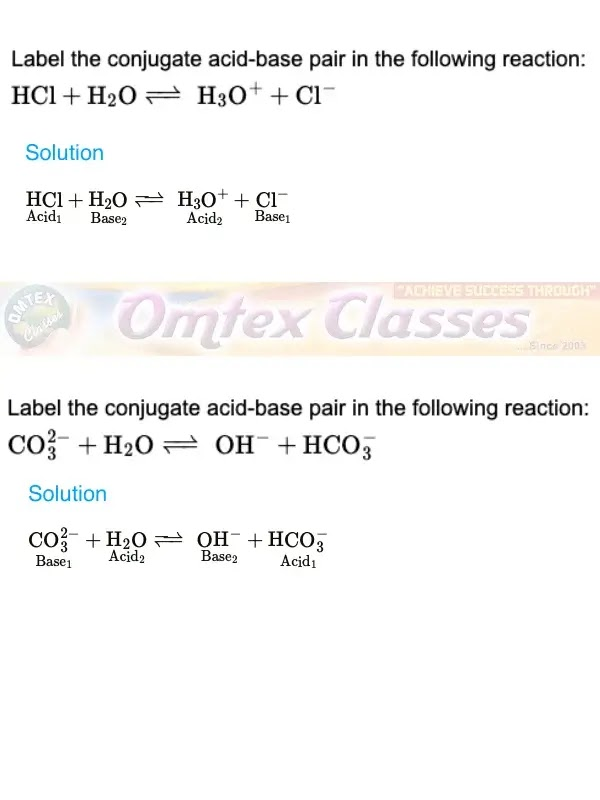Exercises | Q 3.04 | Page 62

Answer the following in brief :

Write a reaction in which water acts as a base.

SOLUTION

Insert Picture Direct.

Exercises | Q 3.05 | Page 62

Answer the following in brief :

Ammonia serves as a Lewis base whereas AlCl3 is Lewis acid. Explain.

SOLUTION

i. According to Lewis's theory, an acid is a substance that can accept a share in an electron pair. In the AlCl3 molecule, the octet of Al is incomplete. Therefore, it can accept an electron pair to complete its octet. Hence, AlCl3 acts as a Lewis acid.

ii. According to Lewis's theory, a base is a substance that can donate an electron pair. In the ammonia (NH3) molecule, the nitrogen atom has one lone pair of electrons to donate. Hence, NH3 acts as a Lewis base.

Exercises | Q 3.06 | Page 62

Answer the following in brief :

Acetic acid is 5% ionised in its decimolar solution. Calculate the dissociation constant of acid.

SOLUTION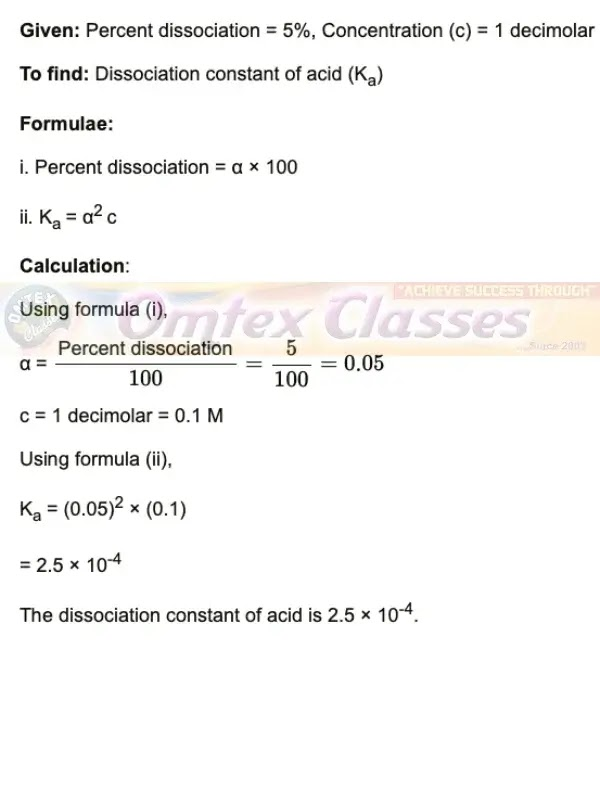Exercises | Q 3.07 | Page 62

Answer the following in brief :

Derive the relation pH + pOH = 14.

SOLUTION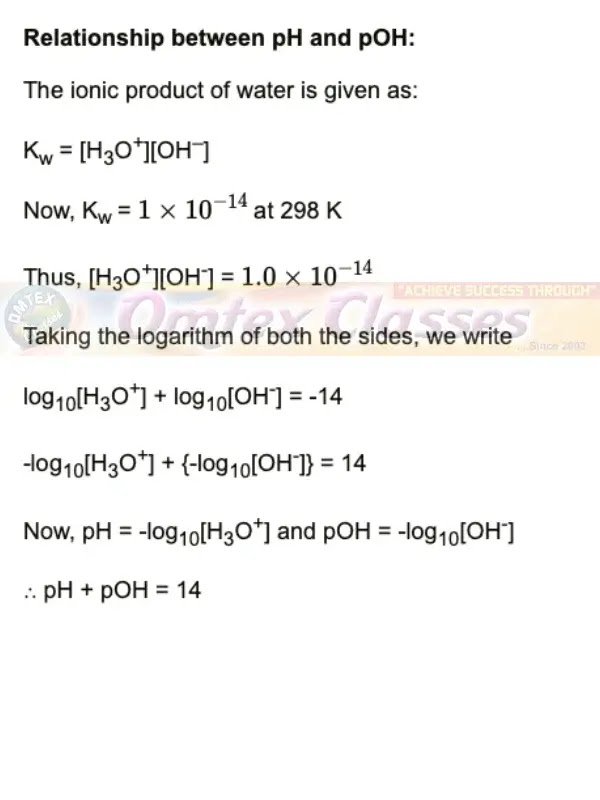Exercises | Q 3.08 | Page 62

Answer the following in brief :

The aqueous solution of sodium carbonate is alkaline whereas the aqueous solution of ammonium chloride is acidic. Explain.

SOLUTION

Exercises | Q 3.09 | Page 62

Answer the following in brief :

The pH of a weak monobasic acid is 3.2 in its 0.02 M solution. Calculate its dissociation constant.

SOLUTION

Exercises | Q 3.1 | Page 62

Answer the following in brief :

In NaOH solution [OH-] is 2.87 × 10-4. Calculate the pH of the solution.

SOLUTION

Exercises | Q 4.01 | Page 62

Define the degree of dissociation

SOLUTION

Exercises | Q 4.01 | Page 62

Derive Ostwald's dilution law for the CH3COOH.

SOLUTION

Exercises | Q 4.02 | Page 62

SOLUTION

Exercises | Q 4.02 | Page 62

SOLUTION

Exercises | Q 4.02 | Page 62

Answer the following in brief :

Derive the relation pH + pOH = 14.

SOLUTION

Exercises | Q 4.03 | Page 62

What is meant by hydrolysis?

SOLUTION

Exercises | Q 4.03 | Page 62

A solution of CH3COONH4 is neutral. why?

SOLUTION

Exercises | Q 4.04 | Page 62

The dissociation of HCN is suppressed by the addition of HCl. Explain.

SOLUTION

Exercises | Q 4.05 | Page 62

Derive the relationship between the degree of dissociation and dissociation constant in weak electrolytes.

SOLUTION

Exercises | Q 4.06 | Page 62

Sulfides of the cation of group II are precipitated in acidic solution (H2S + HCl) whereas sulfides of cations of group IIIB are precipitated in the ammoniacal solution of H2S. Comment on the relative values of the solubility product of sulfides of these.

SOLUTION

Exercises | Q 4.07 | Page 62

The solubility of a sparingly soluble salt gets affected in the presence of a soluble salt having one common ion. Explain.

SOLUTION

Exercises | Q 4.08 | Page 62

The pH of rainwater collected in a certain region of Maharashtra on a particular day was 5.1. Calculate the H+ ion concentration of the rainwater and its percent dissociation.

SOLUTION

Exercises | Q 4.09 | Page 62